Courses

# Sample Test: Quantitative Techniques

## 14 Questions MCQ Test | Sample Test: Quantitative Techniques

Description
This mock test of Sample Test: Quantitative Techniques for CLAT helps you for every CLAT entrance exam. This contains 14 Multiple Choice Questions for CLAT Sample Test: Quantitative Techniques (mcq) to study with solutions a complete question bank. The solved questions answers in this Sample Test: Quantitative Techniques quiz give you a good mix of easy questions and tough questions. CLAT students definitely take this Sample Test: Quantitative Techniques exercise for a better result in the exam. You can find other Sample Test: Quantitative Techniques extra questions, long questions & short questions for CLAT on EduRev as well by searching above.
QUESTION: 1

### Directions: Answer the questions on the basis of the information given below. The break-up of the total number of state government employees in five different states of India - Andhra Pradesh (AP), Gujarat, Uttar Pradesh (UP), Maharashtra and Karnataka in 2018 is depicted in the pie- chart below. The bar graph shows the percentage break-up between permanent and temporary government employees for each state in the same year.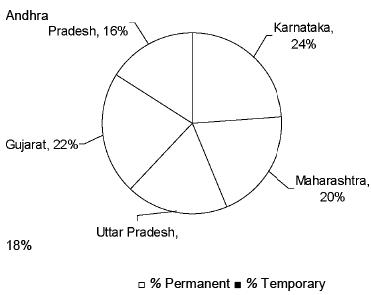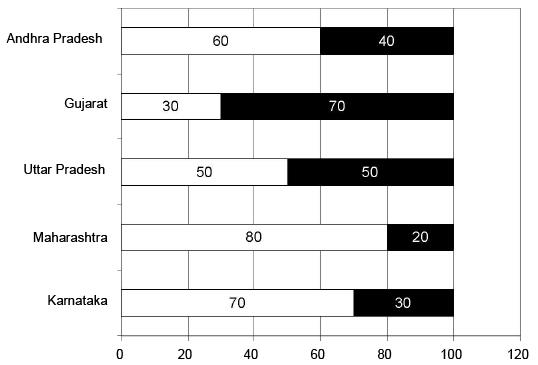If the total number of state government employees in Maharashtra is 9000, then what is the total number of state government employees in Karnataka and Gujarat together?

Solution:

On observing the figures in the table state wise we can see that Uttar Pradesh has more than 17000 units approximately, Assam and Tamil Nadu have more than 13000. On adding the figures for Assam and Tamil Nadu we get the ranking of the top three states as Uttar Pradesh, Assam, Tamil Nadu.

QUESTION: 2

### Directions: Answer the questions on the basis of the information given below. The break-up of the total number of state government employees in five different states of India - Andhra Pradesh (AP), Gujarat, Uttar Pradesh (UP), Maharashtra and Karnataka in 2018 is depicted in the pie- chart below. The bar graph shows the percentage break-up between permanent and temporary government employees for each state in the same year.If Andhra Pradesh has 2880 temporary employees, then what is the total number of employees in Uttar Pradesh?

Solution:

Total number of Micro Units/Enterprises set-up in 2016-17 = 29682
Total number of Micro Units/Enterprises set-up in Uttar Pradesh, Assam and Karnataka together =13677
Required percentage = 29682 * 100 « 46.08%.

QUESTION: 3

### Directions: Answer the questions on the basis of the information given below. The break-up of the total number of state government employees in five different states of India - Andhra Pradesh (AP), Gujarat, Uttar Pradesh (UP), Maharashtra and Karnataka in 2018 is depicted in the pie- chart below. The bar graph shows the percentage break-up between permanent and temporary government employees for each state in the same year.Which two states have the highest and the lowest number of temporary employees respectively?

Solution:

Observing the table we can see that from 2017-18 to 2018-19 Jammu and Kashmir, Chhattisgarh, Gujarat, Karnataka and Tamil Nadu are the only states that show an increase.

QUESTION: 4

Directions: Answer the questions on the basis of the information given below.
The break-up of the total number of state government employees in five different states of India - Andhra Pradesh (AP), Gujarat, Uttar Pradesh (UP), Maharashtra and Karnataka in 2018 is depicted in the pie- chart below. The bar graph shows the percentage break-up between permanent and temporary government employees for each state in the same year.If the states are ranked according to the number of permanent employees, then which two states are first and second respectively?

Solution:

Observing the table we can see that from 2015-16 to 2018-19 only the state of Tamil Nadu shows a continuous increase in the number of Micro Units/ Enterprises set-up under Prime Ministers Employment Generation Programme.

QUESTION: 5

Directions: Answer the questions on the basis of the information given below.
The break-up of the total number of state government employees in five different states of India - Andhra Pradesh (AP), Gujarat, Uttar Pradesh (UP), Maharashtra and Karnataka in 2018 is depicted in the pie- chart below. The bar graph shows the percentage break-up between permanent and temporary government employees for each state in the same year.If the difference between the permanent employees and temporary employees of Karnataka is 192, then what is the total number of permanent employees in Gujarat and Andhra Pradesh together?

Solution:

Average Units/Enterprises in Chhattisgarh 6100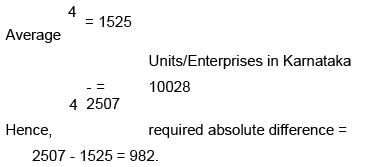QUESTION: 6

Directions: Answer the questions on the basis of the information given below.
A contractor undertakes a project to build a road. It is also known that: 
The length of the road to be built is 15 km. 
Total time allotted for the completion of work is 100 days. 
The contractor employs 50 men for this purpose. 
After 50 days he finds that only 6 km of the road is ready.

How many days behind schedule will the project be if the work continues at the same rate?

Solution:

Let Hitesh's initial speeds in cycling, running and swimming be 6x, 2x and x respectively.
Also after changing his strategy let Hitesh's speeds in cycling, running and swimming be 8y, 3y and y respectively.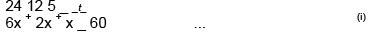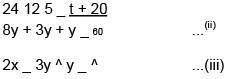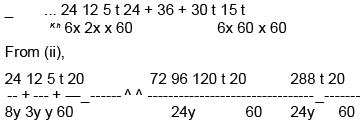Solving the equations we get, x = 9, y = 6 and t = 100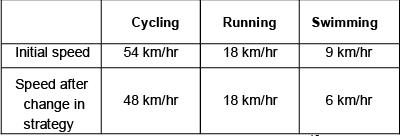Replacing the value of y in (ii) from (iii) we get,18 _ ^60°

Required time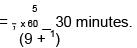QUESTION: 7

Directions: Answer the questions on the basis of the information given below.
A contractor undertakes a project to build a road. It is also known that: 
The length of the road to be built is 15 km. 
Total time allotted for the completion of work is 100 days. 
The contractor employs 50 men for this purpose. 
After 50 days he finds that only 6 km of the road is ready.

What should be the percentage increase in efficiency of the men for the remaining period so that the work is completed as per schedule?

Solution:

Let Hitesh's initial speeds in cycling, running and swimming be 6x, 2x and x respectively.
Also after changing his strategy let Hitesh's speeds in cycling, running and swimming be 8y, 3y and y respectively.Solving the equations we get, x = 9, y = 6 and t = 100Replacing the value of y in (ii) from (iii) we get,18 _ ^60°

Required time = 184 x 60 = 13 minutes.

QUESTION: 8

Directions: Answer the questions on the basis of the information given below.
A contractor undertakes a project to build a road. It is also known that: 
The length of the road to be built is 15 km. 
Total time allotted for the completion of work is 100 days. 
The contractor employs 50 men for this purpose. 
After 50 days he finds that only 6 km of the road is ready.

How many more men should he employ to complete the work as per schedule?

Solution:

Let Hitesh's initial speeds in cycling, running and swimming be 6x, 2x and x respectively.
Also after changing his strategy let Hitesh's speeds in cycling, running and swimming be 8y, 3y and y respectively.Solving the equations we get, x = 9, y = 6 and t = 100Replacing the value of y in (ii) from (iii) we get,18 _ ^60°

Given that Rakesh's speeds during the three phases are in the ratio 4 : 3 : 2 with his running time being same as Hitesh's.
Hence, his speeds in cycling, running and swimming are 24 km/hr, 18 km/hr and 12 km/hr respectively.
Required time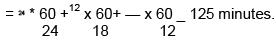QUESTION: 9

Contribution Margin and Market Share of Company A is given for 8 months period. First being April to last being November (simultaneous months from April to November). If the contribution margin of the March is Rs. 1 lakh, then answer the questions based on the data given.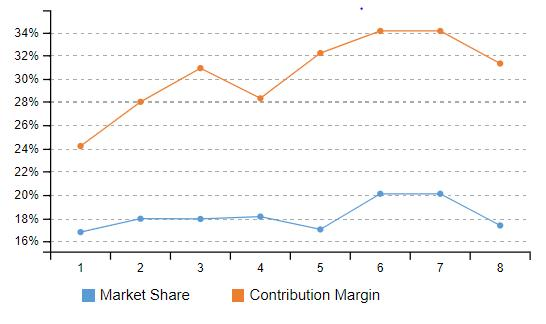How much has market share changed from 4th​​ month to 8th​​ month assuming the customer base is same for all the months?

​​

Solution:

Straight away change in two months Market share calculation. Only subtraction has to be done. Here 4th-month market share is 18.135 while 8th-month market share is 17.36%. The difference is 1.23%.​​

QUESTION: 10

Study the following information and answer the related questions.

P, Q, R, S, T, A, B, C, D and E are employees of a company. A table shows the average age of any two employees of the company. The average age of A and B is 27.5.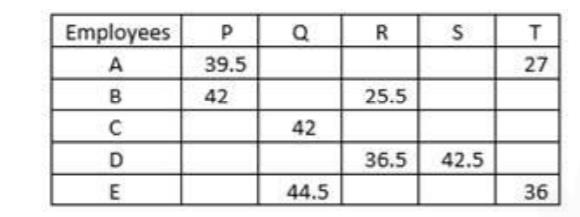i.e. average age of A & P is given as (A+P)/2=39.5

What is the age of T?

Solution:

A + B = 2 X 27.5 = 55

A + P = 2 X 39.5 = 79

B + P = 2 X 42 = 84

A + B + 2P = 79 + 84

2P = 163 – 55

P = 54

Now, A = 79 – 54 = 25

A + T = 2 X 27 = 54

So, T = 54 – 25 = 29

QUESTION: 11

Study the following information and answer the related questions.

P, Q, R, S, T, A, B, C, D and E are employees of a company. A table shows the average age of any two employees of the company. The average age of A and B is 27.5.i.e. average age of A & P is given as (A+P)/2=39.5

What is the average age of D, R and S?

Solution:

B = 55 – 25 = 30

B + R = 2 X 25.5 = 51

So, R = 51 – 30 = 21

D + R = 2 X 36.5 = 73

D = 73 – 21 = 52

And, S + D = 2  X 42.5 = 85

S = 85 – 52 = 33

Therefore, average of D, R and S = 52+21+33 / 3 = 35.33

QUESTION: 12

Study the following information and answer the related questions.

P, Q, R, S, T, A, B, C, D and E are employees of a company. A table shows the average age of any two employees of the company. The average age of A and B is 27.5.i.e. average age of A & P is given as (A+P)/2=39.5

What is the ratio of ages of Q and E?

Solution:

T + E = 2 X 36 = 72

E = 72 – 29 = 43

Q + E = 2 × 44.5 = 89

Q = 89 – 43 = 46

Ratio = 46: 43

QUESTION: 13

Study the following information and answer the related questions.

P, Q, R, S, T, A, B, C, D and E are employees of a company. A table shows the average age of any two employees of the company. The average age of A and B is 27.5.i.e. average age of A & P is given as (A+P)/2=39.5

What will be the average of sum of ages of A, B, C, D and E together after five years?

Solution:

C + Q = 2 × 42 = 84

C = 84 – 46 = 38

Sum of ages of A, B, C, D and E together after five years = (25 + 30 + 38 + 52 + 43) + 25 = 213

Average = 213/5 = 42.6

QUESTION: 14

Study the following information and answer the related questions.

P, Q, R, S, T, A, B, C, D and E are employees of a company. A table shows the average age of any two employees of the company. The average age of A and B is 27.5.i.e. average age of A & P is given as (A+P)/2=39.5

If age of P and Q is decreased by 50% and age of A and B is increased by 20%, what will be the ratio of ages of P, Q, R, S and T together to the ages of A, B, C, D and E together?

Solution:

Age of P and Q is decreased by 50%. So,

New age of P = 50/100 X  54 = 27

New age of Q = 50/100 X 46 = 23

And, age of A and B is increased by 20%. So,

New age of A = 120/100 X 25 = 30

New age of B = 120/100 X 30 = 36

Ratio = 27+ 23+ 21+ 33+ 29 / 30 + 36 + 38 + 52+43   = 133/ 199